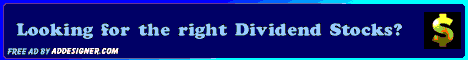Dividends4Life: Dividend Investing + Value Investing = Superior Returns

## Dividend Investing + Value Investing = Superior Returns

Posted by D4L | Thursday, July 10, 2008 | | 0 comments »Some may have wondered what is the connection between Dividend and Value Investing. The connection does not necessarily exist in all cases. For example, an investor selecting investments based solely on dividend yield, is not considering the value aspect of the equation. Conversely, value investors selecting stocks based only on current valuation and future capital appreciation, are ignoring the dividend aspect.

Before going much further, it would be helpful to define value and dividend investing:

Value Investing: As defined by Benjamin Graham and David Dodd generally consists of a system in which the investor identifies market inefficiencies in which a security's price is below its intrinsic value, i.e. it is undervalued. Graham referred to this market price discount from intrinsic value as the "margin of safety". In short, the intrinsic value is the discounted value of all future distributions.

Dividend Investing: In the classic implementation, focuses on identifying solid companies with a record of growing their dividends each year; and an expectation that it will continue into the future. The focus is not solely on yield but a combination of yield and dividend growth. Often it is the lower yield, higher growth, security that will provide the best return over time.

I have always considered Dividend Investing, at least the way I do it, as a subset of Value Investing. Since my first screen is to determine if a stock is a good dividend stock, my universe of stocks is smaller than the average Value Investor. Once a promising dividend stock is located, then I look at its valuation. Is it trading at a discount, fair value or at a premium?

When determining the fair value of a stock I consider four calculations:

1. Avg. High Yield Price: Price calculated by dividing current dividend per share by the average high dividend yield for each of the last 5-years (dividend per share divided by the year's low share price).
2. 20-Year DCF Price: Price calculated by taking the Net Present Value (NPV) of the next 20 years of dividends and the estimated value of the stock at the end of 20 years.
3. Avg. P/E Price: Price calculated by multiplying the EPS (trailing twelve months) times the minimum of: 1.) 5-year average of high and low P/Es or 2.) Last years high P/E.
4. Graham Number: Price calculated by taking the square root of 22.5 times the tangible book value per share times EPS (trailing twelve months). Benjamin Graham, Warren Buffett's mentor and the father of value investing, developed rules for the defensively screening stocks. This formula uses his principles to calculate the "maximum" price one should pay for the stock.
Historically, stocks that pay dividends have out-performed those that don't. When you buy dividend stocks at a discount, it's like turbo-charging your return!

(Photo: Robert Linder)

Related Articles:

________________________________________________________________